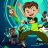# CompoundValue may not work if you use more than 1 in the same study#### korygill

##### Active member
VIP
I ran into an issue where `CompoundValue` does not work if you use more than 1 in the same study. This may not happen all the time, but the workaround I found was to just get rid of that function call, and do the calculations yourself.

You can see the documentation here (that kind of does a poor job describing the function):
https://tlc.thinkorswim.com/center/reference/thinkScript/Functions/Others/CompoundValue

Just use `BarNumber()` and initialize your variable yourself.The 1st parameter is how many bars to initialize your value for (many times, just 1).
The 2nd parameter is the formula once your variable is initialized.
The 3rd parameter is the value to initialize your variable with.

Here's the code so you can test yourself.

Code:
``````declare lower;

input mode = {default UseCompoundValue, ManualCalculation};

def nan = double.NaN;
def bn = BarNumber();

# UseCompoundValue --------------------------------------------------------------------
def x = CompoundValue(2, x + x, 1);
plot FibonacciNumbers1 = if (mode == mode.UseCompoundValue) then x else nan;

# ManualCalculation --------------------------------------------------------------------
def y;
if (bn == 1 or bn == 2) then {
y = 1;
} else {
y = y + y;
}
plot FibonacciNumbers2 = if (mode == mode.ManualCalculation) then y else nan;

And here it is in action!A link so the image above which included the study/code/and layout as shown.

Compound Value Example

Kory Gill, @korygill

•BenTen and diazlaz
R

##### New member
VIP
@korygill - I looked at one of your code using compound value and tried something, but in vain. Could you please help with below?

I am using this code in a watch list column on a 1min time frame.

def ct_5_up = CompoundValue(1, if EMA8 > EMA21 and EMA21 > EMA50 and EMA50 > EMA100 and EMA100 > EMA200 then ct_5_up + 1 else 0, 1);
def ct_3_up = CompoundValue(1, if EMA50 > EMA100 and EMA100 > EMA200 then ct_5_up + 1 else 0, 1);

Basically, I am trying to count the number of bars how long the moving averages are aligned as per the condition above. First I am checking for the ct_5_up and if it is not true, then I am checking next one, ct_3_up. But I want to re-initialize the other one whenever the other condition is true.

It is working fine as expected - but the ct_5_up should be reset to 0, when the ct_3_up condition is met. I tried to use if-else condition but not able to clear the other variable.

Hope I am able to explain what I am trying to achieve and where I am fumbling.

Please suggest. Thank you for your time and help in this regard.

J

#### JoshF

##### New member
Is there a way to rewrite the CompoundValue function such that it would work in a conditional order? Would this manual calculation work?Stocks I'm Watching for the Week of May 27th, 2019 Playground 0